×

Search anything:

# Using if else if statement in C

#### Software Engineering C Programming if elseif else if is a conditional statement that allows a program to execute different code statements based upon a particular value or expression. It is natively supported in C programming language and similarly, in other languages as well.

## if statement in C

The syntax of the if statement in C programming is:

``````if (test expression)
{
/* statements to be executed if
the test expression is true*/
}
``````

#### Flowchart: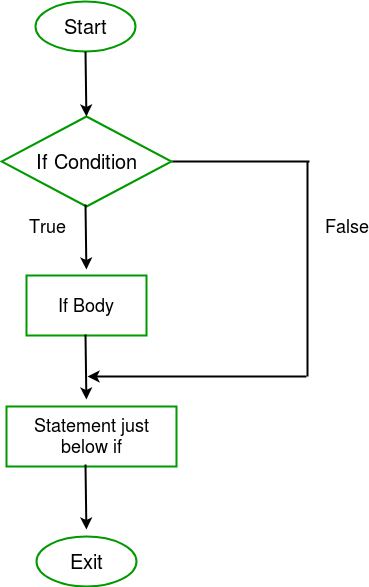#### How if statement works?

The if statement evaluates the test expression inside the parenthesis ().

• If the test expression is evaluated to true, statements inside the body of if are executed.
• If the test expression is evaluated to false, statements inside the body of if are not executed.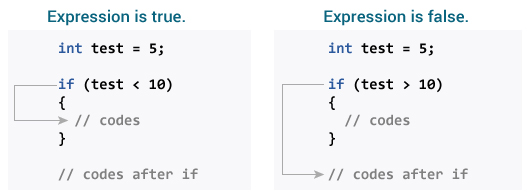#### Example: if statement

``````#include<stdio.h>
using namespace std;

int main()
{
int i = 10;

if (i > 15){
cout<<"10 is less than 15";
}
cout<<"I am Not in if";
}
``````

### Output

``````I am Not in if
``````

## if else Statement in C

The if statement may have an optional else block. The syntax of the if..else statement is:

``````if (test expression) {
/* statements to be executed if the test expression is true */
}
else {
/* statements to be executed if the test expression is false */
}
``````

#### Flowchart: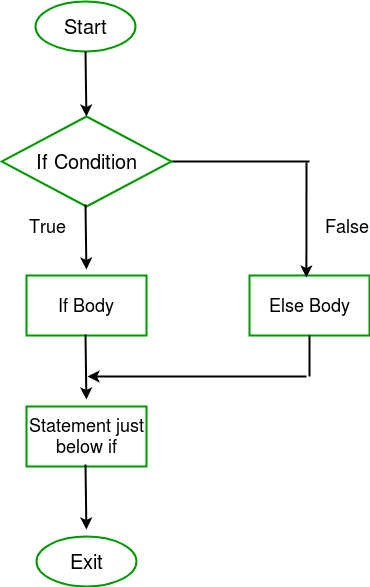#### How if...else statement works?

• If the test expression is evaluated to true,

• statements inside the body of if are executed.
• statements inside the body of else are skipped from execution.
• If the test expression is evaluated to false,

• statements inside the body of else are executed
• statements inside the body of if are skipped from execution.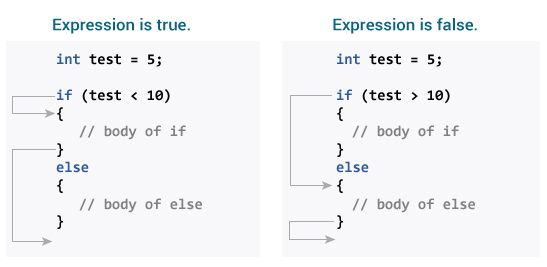#### Example: if...else statement

``````// Check whether an integer is odd or even
#include <stdio.h>
int main()
{
int number;
printf("Enter an integer: ");
scanf("%d", &number);
// True if the remainder is 0
if  (number%2 == 0)
{
printf("%d is an even integer.",number);
}
else
{
printf("%d is an odd integer.",number);
}
return 0;
}
``````

#### Output

``````Enter an integer: 7
7 is an odd integer.
``````

## if else if ladder in C

if else if statements can check for multiple conditions and can take multiple code paths. There are multiple statements to be checked and in a particular order. Once a statement evaluates to true, the rest of the statements are not checked. If no statement (in if and if else) evaluates to true, then the code statements in the else part is executed.

#### Syntax:

``````if (test expression1)
{
// statement(s)
}
else if(test expression2)
{
// statement(s)
}
else if (test  expression3)
{
// statement(s)
}
.
.
else
{
// statement(s)
}
``````

#### Flowchart: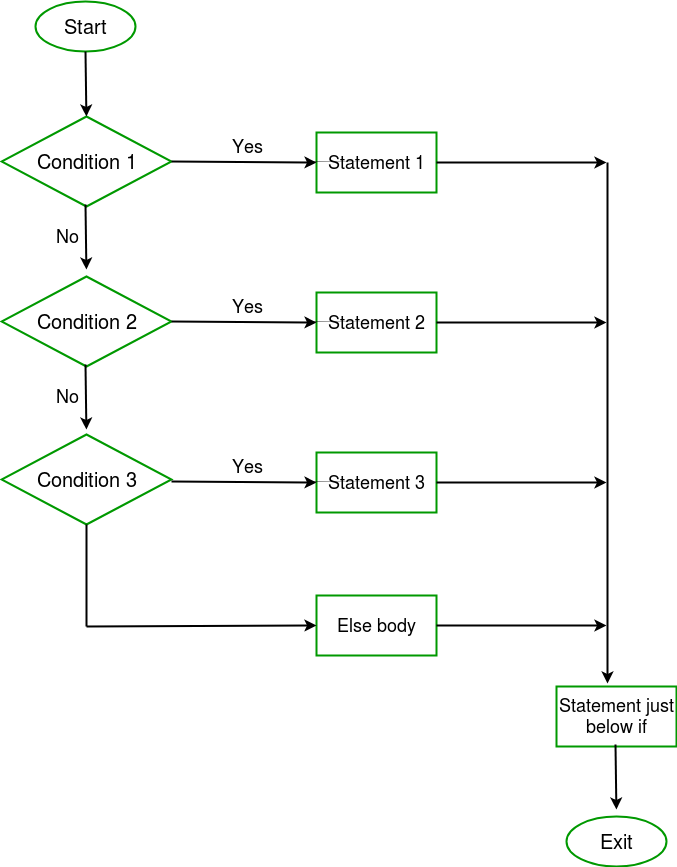``````// Program to relate two integers using =, > or < symbol
#include <stdio.h>
int main()
{
int number1, number2;
printf("Enter two integers: ");
scanf("%d %d", &number1, &number2);
//checks if the two integers are equal.
if(number1 == number2)
{
printf("Result: %d = %d",number1,number2);
}
//checks if number1 is greater than number2.
else if (number1 > number2)
{
printf("Result: %d > %d", number1, number2);
}
//checks if both test expressions are false
else
{
printf("Result: %d < %d",number1, number2);
}
return 0;
}
``````

#### Output

``````Enter two integers: 12
23
Result: 12 < 23
``````

## Nested if else in C

We can place if else statements within if else statements and the nesting can go on to any level.

• It is advised to keep nested if else limited by 2 levels as going beyond it can increase the complexity of the code and make it difficult to understand and more prone to runtime errors.

#### Syntax:

``````if (condition1)
{
// Executes when condition1 is true
if (condition2)
{
// Executes when condition2 is true
}
}
``````

#### Flowchart: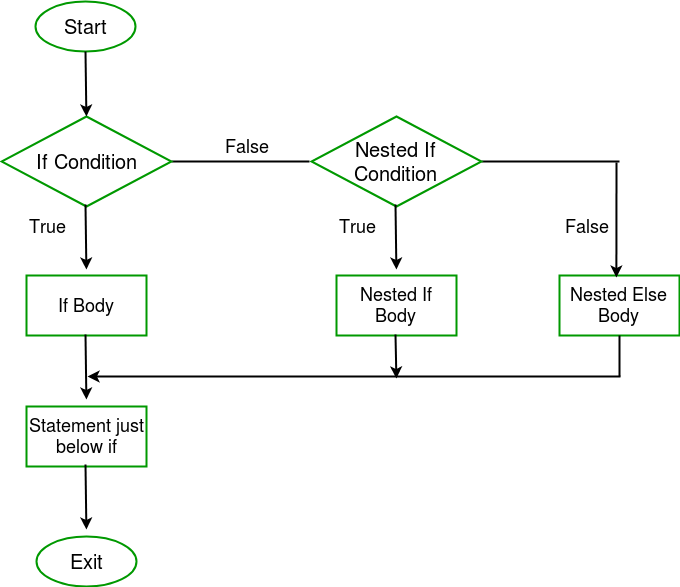#### Example 4: Nested if else

``````// C program to illustrate nested-if statement
int main()
{
int i = 10;

if (i == 10)
{
// First if statement
if (i < 15)
printf("i is smaller than 15");
// Nested - if statement
// Will only be executed if statement above
// it is true
if (i < 12)
printf("i is smaller than 12 too");
else
printf("i is greater than 15");
}

return 0;
}
``````

### Output:

``````i is smaller than 15
i is smaller than 12 too
``````